### Current Electricity Class 12th Physics Part I CBSE Solution

##### Question 1.The earth’s surface has a negative surface charge density of 10-9 C m–2. The potential difference of 400 kV between the top of the atmosphere and the surface results (due to the low conductivity of the lower atmosphere) in a current of only 1800 A over the entire globe. If there were no mechanism of sustaining atmospheric electric field, how much time (roughly) would be required to neutralize the earth’s surface? (This never happens in practice because there is a mechanism to replenish electric charges, namely the continual thunderstorms and lightning in different parts of the globe). (Radius of earth = 6.37 × 106 m.)Answer:Surface Charge density of earth d = 10-9 Coulomb per meter squareCurrent over the entire globe = 1800 AmpereRadius of earth r = 6.37 × 106 meterSurface area of earth A = 4 × π × radius × radiusA = 4 × π × 6.37 × 106 × 6.37 × 106A = 5.09 × 1014 m2Charge on the earth surface q = d × Aq = 10-9 × 5.09 × 1014 m2q = 5.09 × 105 CoulombLet the time taken to neutralize earth surface = tCurrent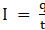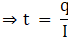t = 5.09 × 105C/1800 At = 282.78 seconds.Question 2.Six lead-acid type of secondary cells each of emf 2.0 V and internal resistance 0.015Ω are joined in series to provide a supply to a resistance of 8.5Ω. What are the current drawn from the supply and its terminal voltage?Answer:Number of secondary cells n = 6Emf of each secondary cell E = 2VoltInternal resistance of each cell r = 0.015 ΩResistance of resistor R = 8.5 ΩLet current drawn from supply = I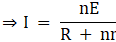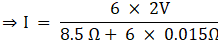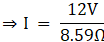⇒ I = 1.39AmpereHence current drawn from supply is 1.39AmpereQuestion 3.A secondary cell after long use has an emf of 1.9 V and a large internal resistance of 380Ω. What maximum current can be drawn from the cell? Could the cell drive the starting motor of a car?Answer:Emf of secondary cell E = 1.9VoltInternal resistance of cell r = 380 Ω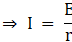⇒ I = 1.9V/380Ω⇒ I = 0.005AmpereHence maximum current drawn from cell is 0.005Ampere and since a large current is required to start the motor of a car and hence cell cannot be used to start the motor.Question 4.Two wires of equal length, one of aluminium and the other of copper have the same resistance. Which of the two wires is lighter? Hence explain why aluminium wires are preferred for overhead power cables. (ρAl = 2.63 × 10–8 Ωm, ρCu = 1.72 × 10–8 Ωm, Relative density of Al = 2.7, of Cu = 8.9.)Answer:Resistivity of aluminium, p1 = 2.63 × 10−8 Ω mRelative density of aluminium, d1 = 2.7Let l1 be the length of aluminium wire and m1 be its mass.Resistance of the aluminium wire = R1Area of cross-section of the aluminium wire = A1Resistivity of copper, p2 = 1.72 × 10−8 Ω metreRelative density of copper, d2 = 8.9Let l2 be the length of copper wire and m2 be its mass.Resistance of the copper wire = R2Area of cross-section of the copper wire = A2We can write,R1 = (p1 × l1)/A1R2 = (p2 × l2)/A2Since R1 = R2(p1 × l1)/A1 = (p2 × l2)/A2And l1 = l2Therefore p1/A1 = p2/A2A1/A2 = (2.63 × 10-8)/(1.72 × 10–8)⇒ A1/A2 = 2.63/1.72Mass of the aluminium wire,m1 = Volume × Density= A1 × l1 × d1 = A1 l1 d1 .....1Mass of the copper wire, m2 = Volume × Density= A2 × l2 × d2 = A2 l2 d2 .......2Dividing equation (1) by equation (2), we obtainm1/m2 = (A1 l1 d1)/(A2 l2 d2)Since l1 = l2m1/m2 = A1 d1/A2 d2Since A1/A2 = 2.63/1.72 calculated above⇒ m1/m2 = (2.63 × 2.7)/(1.72 × 8.9)⇒ m1/m2 = 0.46Since m1 is smaller than m2 Hence, aluminium is lighter than copper.Since aluminium is lighter, it is preferred for overhead power cables over copper.Question 5.What conclusion can you draw from the following observations on a resistor made of alloy manganin?Answer:From the given information shown in the table, the ratio of voltage and current is a constant value and the value is equal to 19.7 Hence manganin is a Ω ic conductor.According to Ohm’s law the ratio of voltage and current is resistance of conductor so Resistance of manganin is 19.7 Ω .Question 6.Answer the following questions:(a) A steady current flows in a metallic conductor of non-uniform cross-section. Which of these quantities is constant along the conductor: current, current density, electric field, drift speed?(b) Is Ohm’s law universally applicable for all conducting elements? If not, give examples of elements which do not obey Ω ’s law.(c) A low voltage supply from which one needs high currents must have very low internal resistance. Why?(d) A high tension (HT) supply of, say, 6 kV must have a very large internal resistance. Why?Answer:(a) When a steady current flows in a metallic conductor of non-uniform cross section, the current flowing through the conductor is constant.Since Current density, electric field and drift speed are inversely proportional to area of cross section therefore they are not constant.(b) No, Ω ’s law is not applicable for all conducting elements. Example of non Ω ic conductor is Vacuum diode semiconductor.(c) According to Ω ’s law V = I × RIf Voltage V is low, then Resistance R must be very low so that high current can be drawn from source.(d) A high Tension supply must have a very large internal resistance so that it can limit the current from exceeding the safety limit.Question 7.Choose the correct alternative:(a) Alloys of metals usually have (greater/less) resistivity than that of their constituent metals.(b) Alloys usually have much (lower/higher) temperature coefficients of resistance than pure metals.(c) The resistivity of the alloy manganin is nearly independent of/ increases rapidly with increase of temperature.(d) The resistivity of a typical insulator (e.g., amber) is greater than that of a metal by a factor of the order of (1022/1023).Answer:(a) Alloys of metals usually have greater resistivity than that of their constituent metals.Alloys are the homogeneous mixture of two or more metals and due to which alloys have better conducting properties as compared to pure metals. Pure metals have large number of free electrons and simple atomic arrangement but in alloys these free electrons number as well as their atomic arrangement changes. Hence alloys have greater resistivity than metals.(b) Alloys usually have lower temperature coefficient of resistance than pure metals.The resistance change factor per °C of temp change is called the temperature coefficient. Coefficient approaching zero can be obtained by alloying certain metals. So we can say that alloys have lower temperature coefficient of resistance than pure metals.(c) The resistivity of alloy, manganin, is nearly independent of increase of temperature.The resistivity of alloys is high and atoms are not arranged in proper order and with the increase in temperature resistivity increases due to collision and random motion of atoms.(d) The resistivity of a typical insulator is greater than that of a metal by a factor of order of 1022.In case of insulator, resistivity decreases with increase of temperature.Question 8.Given n resistors each of resistance R, how will you combine them to get the (i) maximum (ii) minimum effective resistance?What is the ratio of the maximum to minimum resistance?Answer:Total number of resistors = nResistance of each resistor = R(i)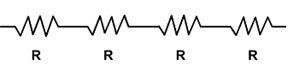When n resistors are connected in series, effective resistance R1is the maximum,given by the product nR.Hence, maximum resistance of the combination, R1 = nR(ii)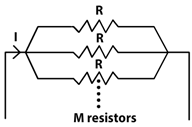When n resistors are connected in parallel, the effective resistance (R2) is the minimum, given by the ratio: R/nHence, minimum resistance of the combination, R2 = R/n(iii) The ratio of the maximum to the minimum resistance is,⇒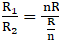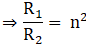Question 9.Given the resistances of 1Ω, 2Ω, 3Ω, how will be combine them to get an equivalent resistance of (i) (11/3) Ω (ii) (11/5) Ω, (iii) 6 Ω, (iv) (6/11) Ω?Answer:(i)The resistance of the given resistors is,R1 = 1 Ω , R2 = 2 Ω , R3 = 3 ΩConnect 2 Ω and 1 Ω resistor in parallel and 3 Ω resistor in series to themEquivalent resistance R’ is {(2 × 1)/(2 + 1)} + 3Hence R’ is equal to 11/3 Ω(ii)Now connect 2 Ω and 3 Ω resistor in parallel and one Ω resistor in series to itEquivalent Resistance R” = {(2 × 3)/(2 + 3)} + 1R” = 11/5 Ω(iii)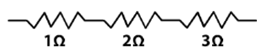Now connect all three resistor 1 Ω 2 Ω and 3 Ω in seriesHence total resistance R”’ is 1 + 2 + 3 = 6 Ω(iv)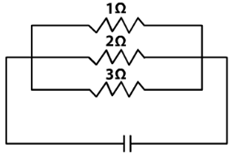Now connect all three resistors in parallel;Equivalent Resistance is R”” = (1 × 2 × 3)/(1 × 2 + 2 × 3 + 3 × 1)R”” = 6/11 ΩQuestion 10.Determine the equivalent resistance of networks shown in Fig. 3.31.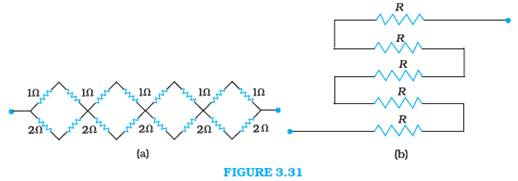Answer:(a)It can be observed from the given circuit that in the first small loop, two resistorsof resistance 1 Ω each are connected in series.Hence, their equivalent resistance = (1 + 1) = 2 ΩIt can also be observed that two resistors of resistance 2 Ω each are connected in series.Hence, their equivalent resistance = (2 + 2) = 4 Ω.Now the given circuit diagram can be explained asNow draw 4 loops each having 2 resistors of 2 Ω and 4 Ω connected in parallel.Hence Equivalent Resistance Re of each loop is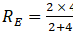⇒ Re = 4/3 ΩSince all 4 loops will be connected in series and each loop will have 4/3 Ω resistanceHence, equivalent resistance of the given circuit is 4 × 4/3 = 16/3 Ω .(b)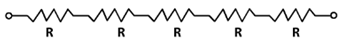From the given circuit that five resistors of resistance R each are connected in series.Hence, equivalent resistance of the circuit = R + R + R + R + R = 5RQuestion 11.Determine the current drawn from a 12V supply with internal resistance 0.5Ω by the infinite network shown in Fig. 3.32. Each resistor has 1Ω resistance.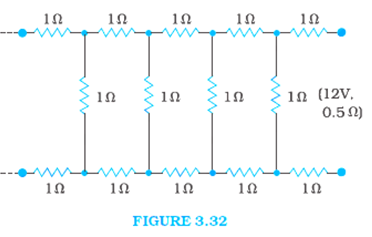Answer:The resistance of each resistor connected in the given circuit, R = 1 ΩEquivalent resistance of the given circuit = R’The network is infinite. Hence, equivalent resistance is given by the relation,R’ = 2 + (R’/R’ + 1)or R’2 -2R’ -2 = 0On solving R’ = (2+√4+8)/2(Negative value of R’ cannot be accepted)R’ = 1 + √3 ΩR’ = 1 + 1.73 = 2.73 ΩInternal resistance of the circuit, r = 0.5 ΩHence, total resistance of the given circuit = 2.73 + 0.5 = 3.23 ΩSupply voltage, V = 12 VAccording to Ω ’s Law, current drawn from the source is given by the ratio, = 12/3.23 = 3.72 AQuestion 12.Figure 3.33 shows a potentiometer with a cell of 2.0 V and internal resistance 0.40 Ω maintaining a potential drop across the resistor wire AB. A standard cell which maintains a constant emf of 1.02 V (for very moderate currents upto a few mA) gives a balance point at 67.3 cm length of the wire. To ensure very low currents drawn from the standard cell, a very high resistance of 600 kΩ is put in series with it, which is shorted close to the balance point. The standard cell is then replaced by a cell of unknown emf e and the balance point found similarly, turns out to be at 82.3 cm length of the wire.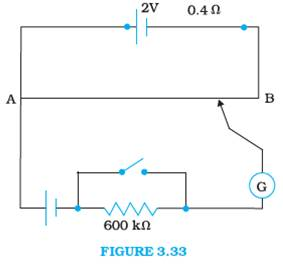(a) What is the value e?(b) What purpose does the high resistance of 600 kW have?(c) Is the balance point affected by this high resistance?(d) Would the method work in the above situation if the driver cell of the potentiometer had an emf of 1.0V instead of 2.0V?(e) Would the circuit work well for determining an extremely small emf, say of the order of a few mV (such as the typical emf of a thermo-couple)? If not, how will you modify the circuit?Answer:(a) Constant emf of the given standard cell, E1 = 1.02 VBalance point on the wire, l1 = 67.3 cmA cell of unknown emf, ε, replaced the standard cell. Therefore, new balance point on the wire,l = 82.3 cmThe relation connecting emf and balance point is,E1/l1 = E/lE = l × E1/l1E = 82.3 cm × 1.02 V/67.3 cmE = 1.247 Volt.The value of unknown emf is 1.247 V.(b) The purpose of using the high resistance of 600 kΩ is to reduce the current through the galvanometer when the movable contact is far from the balance point.(c) The balance point is not affected by the presence of high resistance.(d) The point is not affected by the internal resistance of the driver cell.(e) The method would not work if the driver cell of the potentiometer has an emf of 1.0 V instead of 2.0 V. This is because if the emf of the driver cell of the potentiometer is less than the emf of the other cell, then we cannot obtain balance point on the wire.(f) The circuit would not work well for determining an extremely small emf. As the circuit would be unstable, the balance point would be close to end A. Hence, there would be a high percentage of error. The given circuit can be modified if a series resistance is connected with the wire AB. The potential drop across AB is slightly greater than the emf measured. The percentage error would be small.Question 13.Figure 3.34 shows a 2.0 V potentiometer used for the determination of internal resistance of a 1.5 V cell. The balance point of the cell in open circuit is 76.3 cm. When a resistor of 9.5Ω is used in the external circuit of the cell, the balance point shifts to 64.8 cm length of the potentiometer wire. Determine the internal resistance of the cell.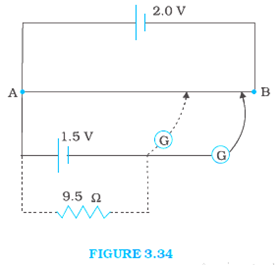Answer:Balance point for open circuit, l1 = 78.3 cmResistance of the external resistor, R = 9.5 ΩBalance point for this resistor, l2 = 64.8 cmThe relation connecting internal resistance r and balance point is,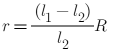r=(78.3-64.8)/64.8× 9.5=0.177 × 9.5=1.685 ΩTherefore, the value of the unknown resistance, X, is 1.685 Ω.

PDF FILE TO YOUR EMAIL IMMEDIATELY PURCHASE NOTES & PAPER SOLUTION. @ Rs. 50/- each (GST extra)

HINDI ENTIRE PAPER SOLUTION

MARATHI PAPER SOLUTION

SSC MATHS I PAPER SOLUTION

SSC MATHS II PAPER SOLUTION

SSC SCIENCE I PAPER SOLUTION

SSC SCIENCE II PAPER SOLUTION

SSC ENGLISH PAPER SOLUTION

SSC & HSC ENGLISH WRITING SKILL

HSC ACCOUNTS NOTES

HSC OCM NOTES

HSC ECONOMICS NOTES

HSC SECRETARIAL PRACTICE NOTES

# 2019 Board Paper Solution

HSC ENGLISH SET A 2019 21st February, 2019

HSC ENGLISH SET B 2019 21st February, 2019

HSC ENGLISH SET C 2019 21st February, 2019

HSC ENGLISH SET D 2019 21st February, 2019

SECRETARIAL PRACTICE (S.P) 2019 25th February, 2019

HSC XII PHYSICS 2019 25th February, 2019

CHEMISTRY XII HSC SOLUTION 27th, February, 2019

OCM PAPER SOLUTION 2019 27th, February, 2019

HSC MATHS PAPER SOLUTION COMMERCE, 2nd March, 2019

HSC MATHS PAPER SOLUTION SCIENCE 2nd, March, 2019

SSC ENGLISH STD 10 5TH MARCH, 2019.

HSC XII ACCOUNTS 2019 6th March, 2019

HSC XII BIOLOGY 2019 6TH March, 2019

HSC XII ECONOMICS 9Th March 2019

SSC Maths I March 2019 Solution 10th Standard11th, March, 2019

SSC MATHS II MARCH 2019 SOLUTION 10TH STD.13th March, 2019

SSC SCIENCE I MARCH 2019 SOLUTION 10TH STD. 15th March, 2019.

SSC SCIENCE II MARCH 2019 SOLUTION 10TH STD. 18th March, 2019.

SSC SOCIAL SCIENCE I MARCH 2019 SOLUTION20th March, 2019

SSC SOCIAL SCIENCE II MARCH 2019 SOLUTION, 22nd March, 2019

XII CBSE - BOARD - MARCH - 2019 ENGLISH - QP + SOLUTIONS, 2nd March, 2019

# HSCMaharashtraBoardPapers2020

(Std 12th English Medium)

HSC ECONOMICS MARCH 2020

HSC OCM MARCH 2020

HSC ACCOUNTS MARCH 2020

HSC S.P. MARCH 2020

HSC ENGLISH MARCH 2020

HSC HINDI MARCH 2020

HSC MARATHI MARCH 2020

HSC MATHS MARCH 2020

# SSCMaharashtraBoardPapers2020

(Std 10th English Medium)

English MARCH 2020

HindI MARCH 2020

Hindi (Composite) MARCH 2020

Marathi MARCH 2020

Mathematics (Paper 1) MARCH 2020

Mathematics (Paper 2) MARCH 2020

Sanskrit MARCH 2020

Sanskrit (Composite) MARCH 2020

Science (Paper 1) MARCH 2020

Science (Paper 2)

Geography Model Set 1 2020-2021

MUST REMEMBER THINGS on the day of Exam

Are you prepared? for English Grammar in Board Exam.

Paper Presentation In Board Exam

How to Score Good Marks in SSC Board Exams

Tips To Score More Than 90% Marks In 12th Board Exam

How to write English exams?

How to prepare for board exam when less time is left

How to memorise what you learn for board exam

No. 1 Simple Hack, you can try out, in preparing for Board Exam

How to Study for CBSE Class 10 Board Exams Subject Wise Tips?

JEE Main 2020 Registration Process – Exam Pattern & Important Dates

NEET UG 2020 Registration Process Exam Pattern & Important Dates

How can One Prepare for two Competitive Exams at the same time?

8 Proven Tips to Handle Anxiety before Exams!